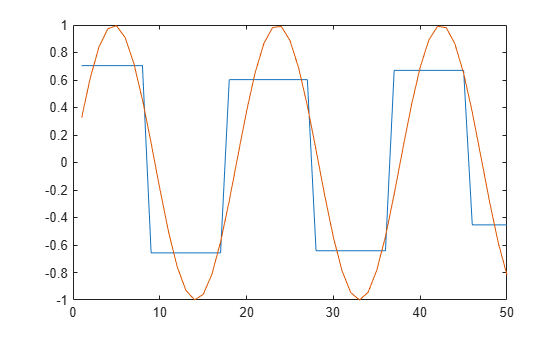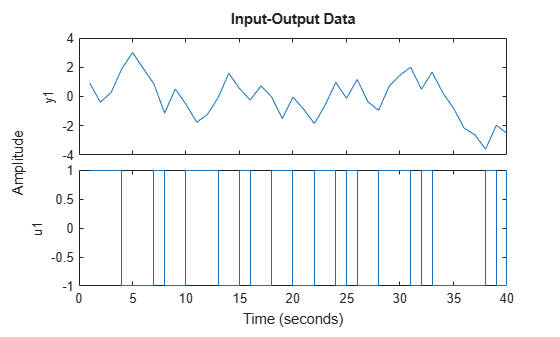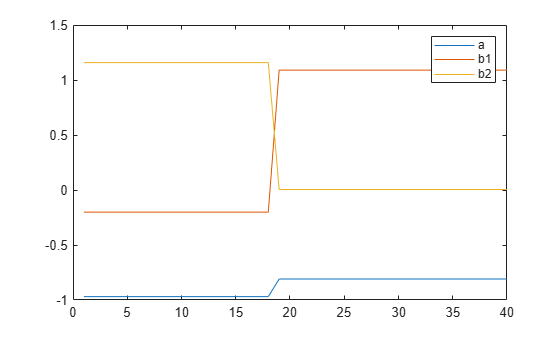Documentation

# segment

Segment data and estimate models for each segment

## Syntax

```segm = segment(z,nn)
[segm,V,thm,R2e] = segment(z,nn,R2,q,R1,M,th0,P0,ll,mu)
```

## Description

`segment` is not compatible with MATLAB® Coder™ or MATLAB Compiler™.

`segment` builds models of AR, ARX, or ARMAX/ARMA type,

`$A\left(q\right)y\left(t\right)=B\left(q\right)u\left(t-nk\right)+C\left(q\right)e\left(t\right)$`

assuming that the model parameters are piecewise constant over time. It results in a model that has split the data record into segments over which the model remains constant. The function models signals and systems that might undergo abrupt changes.

The input-output data is contained in `z`, which is either an `iddata` object or a matrix ```z = [y u]``` where `y` and `u` are column vectors. If the system has several inputs, `u` has the corresponding number of columns.

The argument `nn` defines the model order. For the ARMAX model

```nn = [na nb nc nk]; ```

where `na`, `nb`, and `nc` are the orders of the corresponding polynomials. See What Are Polynomial Models?. Moreover, `nk` is the delay. If the model has several inputs, `nb` and `nk` are row vectors, giving the orders and delays for each input.

For an ARX model (`nc = 0`) enter

```nn = [na nb nk]; ```

For an ARMA model of a time series

```z = y; nn = [na nc]; ```

and for an AR model

```nn = na; ```

The output argument `segm` is a matrix, where the `k`th row contains the parameters corresponding to time `k`. This is analogous to output estimates returned by the `recursiveARX` and `recursiveARMAX` estimators. The output argument `thm` of `segment` contains the corresponding model parameters that have not yet been segmented. Each row of `thm` contains the parameter estimates at the corresponding time instant. These estimates are formed by weighting together the parameters of `M` (default: 5) different time-varying models, with the participating models changing at every time step. Consider `segment` as an alternative to the online estimation commands when you are not interested in continuously tracking the changes in parameters of a single model, but need to detect abrupt changes in the system dynamics.

The output argument `V` contains the sum of the squared prediction errors of the segmented model. It is a measure of how successful the segmentation has been.

The input argument `R2` is the assumed variance of the innovations e(t) in the model. The default value of `R2`, ```R2 = []```, is that it is estimated. Then the output argument `R2e` is a vector whose `k`th element contains the estimate of `R2` at time `k`.

The argument `q` is the probability that the model exhibits an abrupt change at any given time. The default value is `0.01`.

`R1` is the assumed covariance matrix of the parameter jumps when they occur. The default value is the identity matrix with dimension equal to the number of estimated parameters.

`M` is the number of parallel models used in the algorithm (see below). Its default value is `5`.

`th0` is the initial value of the parameters. Its default is zero. `P0` is the initial covariance matrix of the parameters. The default is 10 times the identity matrix.

`ll` is the guaranteed life of each of the models. That is, any created candidate model is not abolished until after at least `ll` time steps. The default is ```ll = 1```. `Mu` is a forgetting parameter that is used in the scheme that estimates `R2`. The default is `0.97`.

The most critical parameter for you to choose is `R2`. It is usually more robust to have a reasonable guess of `R2` than to estimate it. Typically, you need to try different values of `R2` and evaluate the results. (See the example below.) `sqrt(R2)` corresponds to a change in the value y(t) that is normal, giving no indication that the system or the input might have changed.

## Examples

collapse all

Create a sinusoid for the simulated model output.

`y = sin([1:50]/3)';`

Specify the input signal to be constant at `1`.

`u = ones(size(y));`

Specify the estimated noise variance for the model.

`R2 = 0.1;`

Segment the signal and estimate an ARX model for each segment. Use the simple model $y\left(t\right)={b}_{1}u\left(t-1\right)$, where $b1$ is the model parameter describing the piecewise constant level of the estimated output, $y\left(t\right)$.

`segm = segment([y,u],[0 1 1],R2);`

Examine the result.

`plot([segm,y])`Vary the value of `R2` to change the estimated noise variance. Decreasing `R2` increases the number of segments produced for this model.

Load and plot the estimation data.

```load iddemo6m.mat z z = iddata(z(:,1),z(:,2)); plot(z)```This data contains a change in time delay from `2` to `1`, which is difficult to detect by examining the data.

Specify the model orders to estimate an ARX model of the form:

`$y\left(t\right)+ay\left(t-1\right)={b}_{1}u\left(t-1\right)+{b}_{2}u\left(t-2\right)$`

`nn = [1 2 1];`

Segment the data and estimate ARX models for each segment. Specify an estimated noise variance of `0.1`.

`seg = segment(z,nn,0.1);`

Examine the parameters of the segmented model.

```plot(seg) legend('a','b1','b2');```The data has been divided into two segments, as indicated by the change in model parameters around sample number 19. The increase in `b1`, along with a corresponding decrease in `b2`, shows the change in model delay.

## Algorithms

The algorithm is based on `M` parallel models, each recursively estimated by an algorithm of Kalman filter type. Each model is updated independently, and its posterior probability is computed. The time-varying estimate `thm` is formed by weighting together the `M` different models with weights equal to their posterior probability. At each time step the model (among those that have lived at least `ll` samples) that has the lowest posterior probability is abolished. A new model is started, assuming that the system parameters have changed, with probability `q`, a random jump from the most likely among the models. The covariance matrix of the parameter change is set to `R1`.

After all the data are examined, the surviving model with the highest posterior probability is tracked back and the time instances where it jumped are marked. This defines the different segments of the data. (If no models had been abolished in the algorithm, this would have been the maximum likelihood estimates of the jump instances.) The segmented model `segm` is then formed by smoothing the parameter estimate, assuming that the jump instances are correct. In other words, the last estimate over a segment is chosen to represent the whole segment.